×

Search anything:

# Convert Binary to Hexadecimal, Octal, Decimal

#### Algorithms List of Mathematical AlgorithmsGet this book -> Problems on Array: For Interviews and Competitive Programming

In this article, we will discuss how we can convert a binary number to a hexadecimal, octal, and decimal number.

1. How to convert from Binary to Hexadecimal
2. How to convert from Binary to Octal
3. How to convert from Binary to Decimal

Let us get started with discussing how different types of numerical systems such as hexadecmial, octal, and decimal can be obtained from a binary number through conversion.

### How to convert from Binary to Hexadecimal?

Step 1: Seperate your binary number into groups of 4.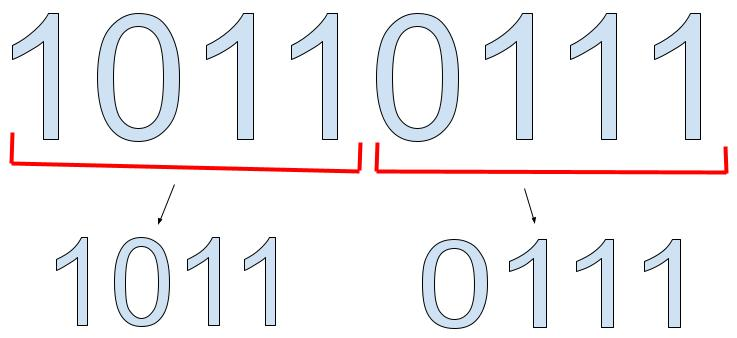Step 2: Now we have to convert the binary numbers to decimal values.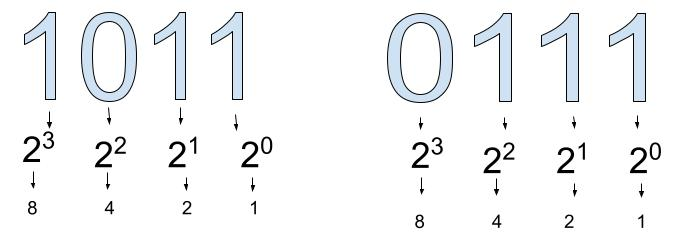Step 3: Multiply the decimal values by their binary number and add them all up.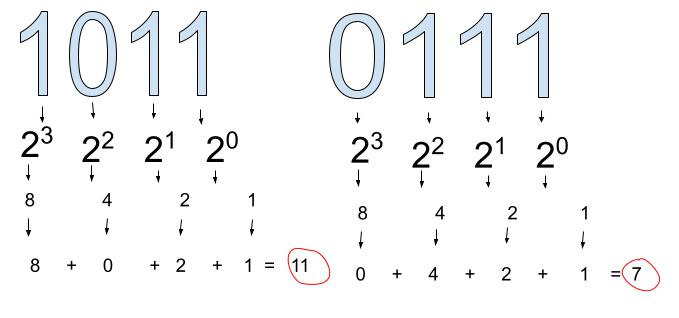Step 4: Now we can convert the numbers we have obtained to hexadecimal values using the following hexadecimal chart. From the chart we can see that the number 7 corresponds to "7" on the chart while the number 11 corresponds to "B". Since hexadecimals are a base 16 system. We put 16 after B7 using subscript.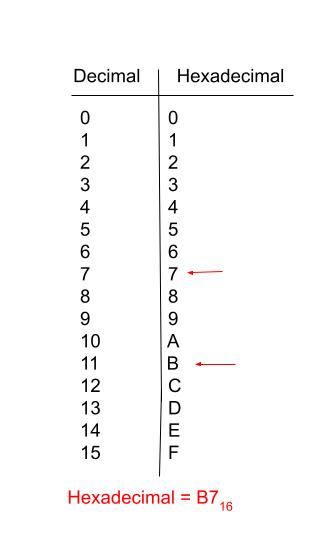Now let us discuss how we can create a program consisting of pseudocode and implementation on how to convert binary to hexidecimal.

``````# Convert from Binary to decimal and then convert from decimal to hexadecimal (process)
# Create an list called hexa that is blank.
hexa = []
# Create a variable called result that will print the reversed hexadecimal.
result = []
# create a variable called num that will be the number input from the user.
num = int(input("Enter any number: "))
# Create a variable called des to determine the sum of the decimal value.
des = 0
# Create a variable called i that will be the index of the list.
i = 0
# Create a variable called temp to copy the num variable value.
temp = num
# Create a while loop that will run as long as the temp variable is greater than 0.
while temp > 0:
# Create a variable called rem that will be the remainder of the temp % 10
rem = int(temp % 10)
# Create a variable called des that will be the sum of the des variable and the rem variable.
des = des + rem * pow(2, i)
# Increment i by 1 each time the loop runs. This will be the index of the list. To move on to the next element in the list.
i = i + 1
# Divide the temp variable by 10 to get the next digit. That will go through the loop again.
temp = int(temp / 10)
# Now we have convert our number to decimal. We can print it to confirm. We can felete this after.
print("Decimal: ", des)
# Set i = 0` to start the loop again and interate through the decimal value.
i = 0
# Create a variable called rem that will be the remainder.
rem = 0
# Create a while loop that will run as long as the des variable is greater than 0.
while des > 0:
# Since hexadecimal is base 16, we can use the des % 16 to get the remainder.
rem = int(des % 16)
# Check if the remainder is between 0 and 9 or between 10 and 15.
if rem < 10:
# If the remainder is between 0 and 9, we can add the remainder to the list.
hexa.append(chr(rem + 48))
else:
# If the remainder is between 10 and 15, we can add the remainder to the list.
hexa.append(chr(rem + 55))
# Then we can decrement the divide des variable by 16 to get the next remainder.
des = int(des / 16)
# Start the loop again to reverse the list. This is 1.
j = 1
# For loop to interate through the list. Starting from i.
for i in hexa:
# Append the reversed list to the result variable.
result.append(hexa[len(hexa) - j])
# Now we can increment j by 1 each time the loop runs.
j = j + 1
print(result)
``````

Time Complexity = O(N), dependent on user input size.

### How to convert from Binary to Octal?

Step 1: separate binary number into groups of 3.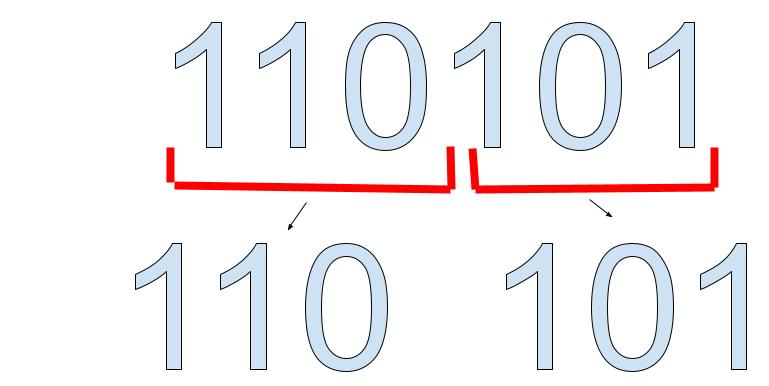Step 2: Multiply each digit in the group of 3 by the numbers under them.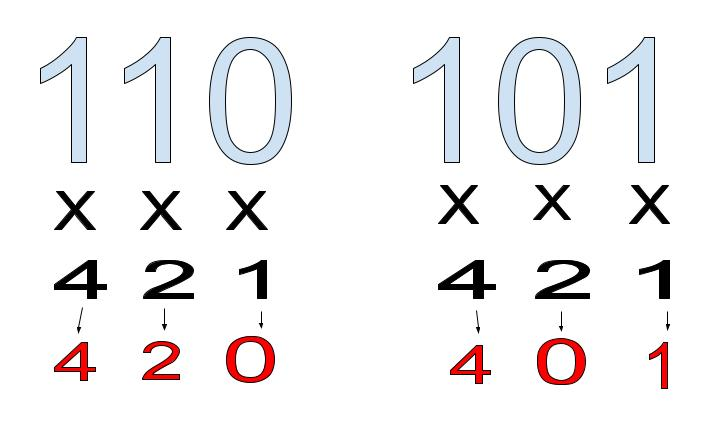Step 3: Add the numbers in the two groups up and combine them. Put an 8 after the number in subscript to make it in the base 8 system which is octal.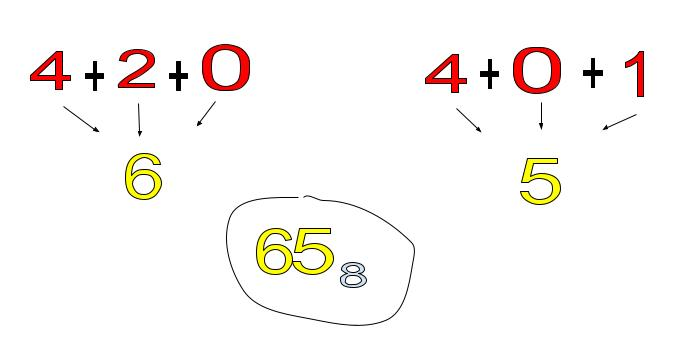Now let us discuss how we can create a program consisting of pseudocode and implementation on how to convert binary to octal.

``````# Create a list with a binary number and store it in num variable.
num = 
# Create a variable called oct_num and set its value to 0.
oct_num = 0
# Create a variable called dec_num and set its value to 0.
dec_num = 0
# Create a variable called a and set its value to 0.
a = 0
# Loop until the input binary number is not equal to zero using a while loop.
print(f"The Octal value binary number {num} is: ")
while (num != 0):
# Inside the loop, get last digit using modulus operator and use value of 10
# Create a variable called b and store it in the value of the modulus operator.
b = (num % 10)
# Get the value of 2 raised to the power of a using the pow() function and multiply
# it with the  value b from above.
# Store it in a variable called c
c = pow(2, a) * b
# Add c value to the dec_num variable and store it in the same variable dec_num.
dec_num = dec_num + c
# Increase the value a by 1 and store in variable a.
a += 1
# Divide the given binary number by 10 and store it in the  variable num.
num = num // 10
# exit while loop and set the value of variable with 1.
a = 1
# Loop until the decimal number is not equal to zero from a while loop.
while (dec_num != 0):
# Multiply variable a value with the num modulus 8 and store it in a variable called d. This will give the octal value.
d = (dec_num % 8) * a
# Add the value of d with the oct_num  variable and store it in that variable.
# variable oct_num.
oct_num = oct_num + d
# Divide the dec_num by 8 and store it  in dec_num variable.
dec_num = dec_num // 8
# Multiply a with 10 and store it iun a variable called a.
a = a * 10
# Print the oct_num to finish program. .
print(oct_num)
``````

Time Complexity = O(N), dependent on user input size.

### How to convert from Binary to decimal?

Step 1: Multiply each digit in the binary number by its corresponding powers of two.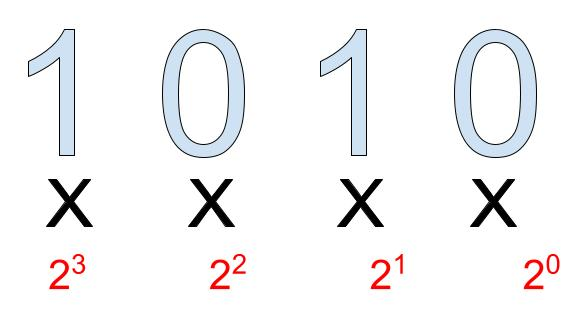Step 2: Add all of the values to calculate your decimal number.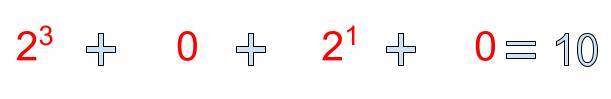Now let us discuss how we can create a program consisting of pseudocode and implementation on how to convert binary to decimal.

``````# Convert from Binary to decimal
# Create an list called hexa that is blank.
hexa = []
# Create a variable called result that will print the reversed hexadecimal.
result = []
# create a variable called num that will be the number input from the user.
num = int(input("Enter any number: "))
# Create a variable called des to determine the sum of the decimal value.
des = 0
# Create a variable called i that will be the index of the list.
i = 0
# Create a variable called temp to copy the num variable value.
temp = num
# Create a while loop that will run as long as the temp variable is greater than 0.
while temp > 0:
# Create a variable called rem that will be the remainder of the temp % 10
rem = int(temp % 10)
# Create a variable called des that will be the sum of the des variable and the rem variable.
des = des + rem * pow(2, i)
# Increment i by 1 each time the loop runs. This will be the index of the list. To move on to the next element in the list.
i = i + 1
# Divide the temp variable by 10 to get the next digit. That will go through the loop again.
temp = int(temp / 10)
# Now we have convert our number to decimal. We can print it to confirm. We can felete this after.

print("Decimal: ", des)

``````

Time Complexity = O(N), dependent on user input size.

### Real life applications

1. While computer networks and devices use binary-based addressing, humans utilize decimal-based addressing. We can receive information in a language that is simple for us to understand thanks to conversions.

## Question

#### What is the binary number 1010 in decimal form?

10
23
4
7
Using the above mentioned conversion technique. We can multiply each digit in the binary number with its corresponding power of 2. We then add all of the numbers up to get 10.